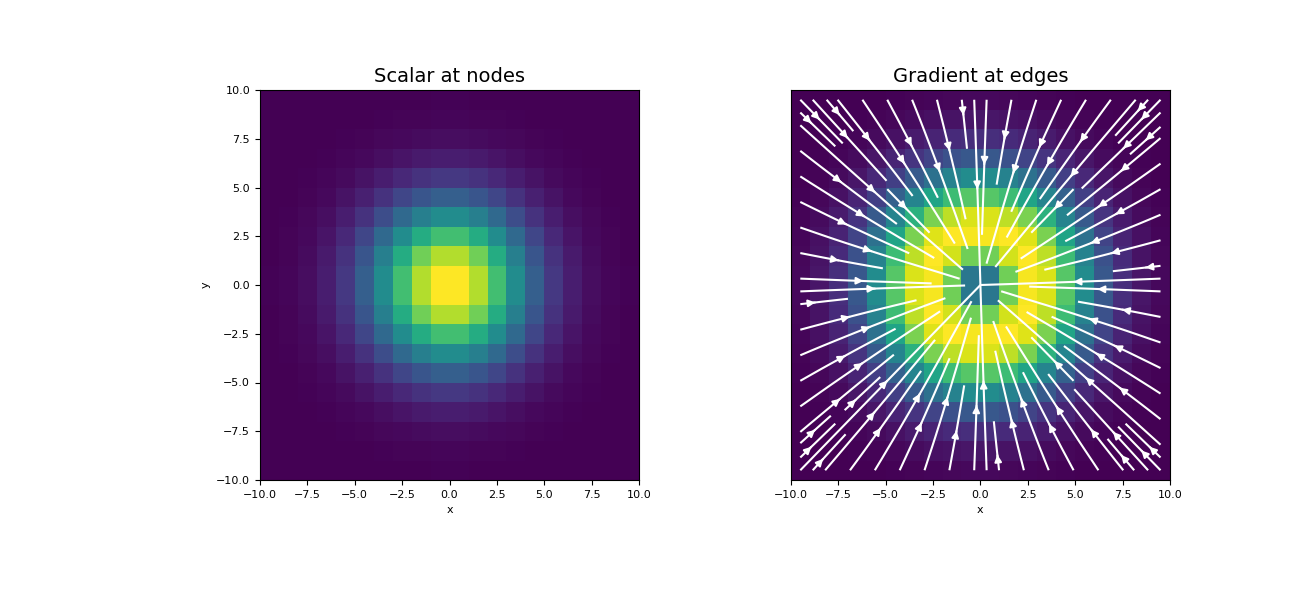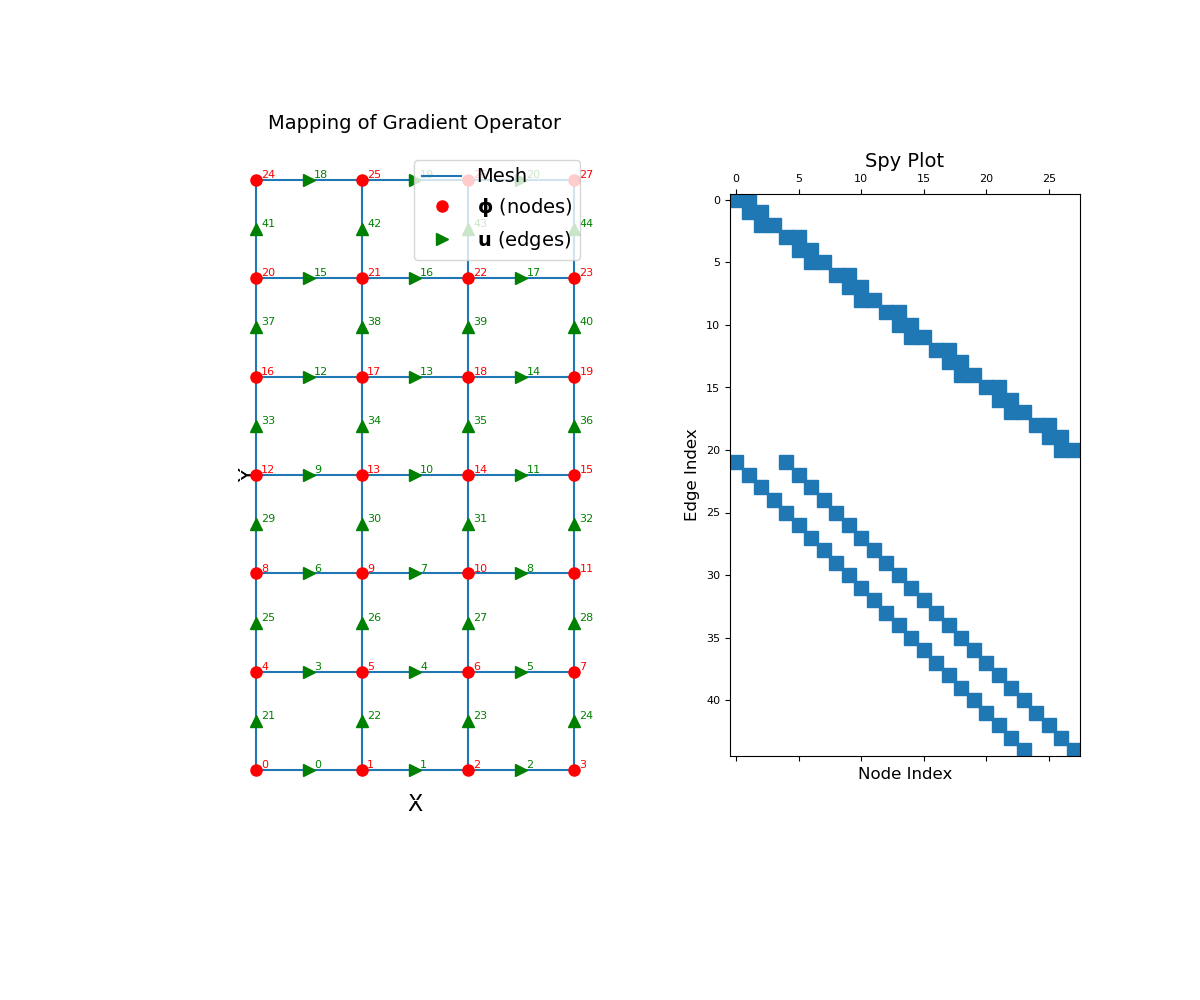Nodal gradient operator (nodes to edges)

This property constructs the 2nd order numerical gradient operator that maps from nodes to edges. The operator is a sparse matrix $$\mathbf{G_n}$$ that can be applied as a matrix-vector product to a discrete scalar quantity $$\boldsymbol{\phi}$$ that lives on the nodes, i.e.:

grad_phi = Gn @ phi


Once constructed, the operator is stored permanently as a property of the mesh.

Returns
(n_edges, n_nodes) scipy.sparse.csr_matrix

The numerical gradient operator from nodes to edges

Notes

In continuous space, the gradient operator is defined as:

$\vec{u} = \nabla \phi = \frac{\partial \phi}{\partial x}\hat{x} + \frac{\partial \phi}{\partial y}\hat{y} + \frac{\partial \phi}{\partial z}\hat{z}$

Where $$\boldsymbol{\phi}$$ is the discrete representation of the continuous variable $$\phi$$ on the nodes and $$\mathbf{u}$$ is the discrete representation of $$\vec{u}$$ on the edges, nodal_gradient constructs a discrete linear operator $$\mathbf{G_n}$$ such that:

$\mathbf{u} = \mathbf{G_n} \, \boldsymbol{\phi}$

The Cartesian components of $$\vec{u}$$ are defined on their corresponding edges (x, y or z) as follows; e.g. the x-component of the gradient is defined on x-edges. For edge $$i$$ which defines a straight path of length $$h_i$$ between adjacent nodes $$n_1$$ and $$n_2$$:

$u_i = \frac{\phi_{n_2} - \phi_{n_1}}{h_i}$

Note that $$u_i \in \mathbf{u}$$ may correspond to a value on an x, y or z edge. See the example below.

Examples

Below, we demonstrate 1) how to apply the nodal gradient operator to a discrete scalar quantity, and 2) the mapping of the nodal gradient operator and its sparsity. Our example is carried out on a 2D mesh but it can be done equivalently for a 3D mesh.

We start by importing the necessary packages and modules.

>>> from discretize import TensorMesh
>>> import numpy as np
>>> import matplotlib.pyplot as plt
>>> import matplotlib as mpl


For a discrete scalar quantity defined on the nodes, we take the gradient by constructing the gradient operator and multiplying as a matrix-vector product.

Create a uniform grid

>>> h = np.ones(20)
>>> mesh = TensorMesh([h, h], "CC")


Create a discrete scalar on nodes

>>> nodes = mesh.nodes
>>> phi = np.exp(-(nodes[:, 0] ** 2 + nodes[:, 1] ** 2) / 4 ** 2)


Construct the gradient operator and apply to vector

>>> Gn = mesh.nodal_gradient
>>> grad_phi = Gn @ phi


Plot the original function and the gradient

Expand to show scripting for plot
>>> fig = plt.figure(figsize=(13, 6))
>>> mesh.plot_image(phi, v_type="N", ax=ax1)
>>> ax1.set_title("Scalar at nodes", fontsize=14)
>>> mesh.plot_image(
...     stream_opts={"color": "w", "density": 1.0}
... )
>>> ax2.set_yticks([])
>>> ax2.set_ylabel("")
>>> plt.show()The nodal gradient operator is a sparse matrix that maps from nodes to edges. To demonstrate this, we construct a small 2D mesh. We then show the ordering of the elements in the original discrete quantity $$\boldsymbol{\phi}$$ and its discrete gradient as well as a spy plot.

Expand to show scripting for plot
>>> mesh = TensorMesh([[(1, 3)], [(1, 6)]])
>>> fig = plt.figure(figsize=(12, 10))
>>> mesh.plot_grid(ax=ax1)
>>> ax1.plot(mesh.nodes[:, 0], mesh.nodes[:, 1], "ro", markersize=8)
>>> for ii, loc in zip(range(mesh.nN), mesh.nodes):
>>>     ax1.text(loc + 0.05, loc + 0.02, "{0:d}".format(ii), color="r")

>>> ax1.plot(mesh.edges_x[:, 0], mesh.edges_x[:, 1], "g>", markersize=8)
>>> for ii, loc in zip(range(mesh.nEx), mesh.edges_x):
>>>     ax1.text(loc + 0.05, loc + 0.02, "{0:d}".format(ii), color="g")

>>> ax1.plot(mesh.edges_y[:, 0], mesh.edges_y[:, 1], "g^", markersize=8)
>>> for ii, loc in zip(range(mesh.nEy), mesh.edges_y):
>>>     ax1.text(loc + 0.05, loc + 0.02, "{0:d}".format((ii + mesh.nEx)), color="g")

>>> ax1.set_xticks([])
>>> ax1.set_yticks([])
>>> ax1.spines['bottom'].set_color('white')
>>> ax1.spines['top'].set_color('white')
>>> ax1.spines['left'].set_color('white')
>>> ax1.spines['right'].set_color('white')
>>> ax1.legend(
>>>     ['Mesh', '$\mathbf{\phi}$ (nodes)', '$\mathbf{u}$ (edges)'],
>>>     loc='upper right', fontsize=14
>>> )